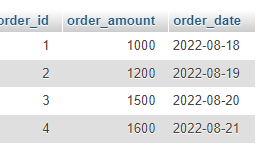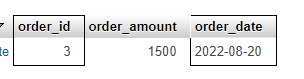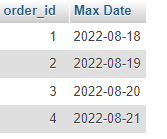# MySQL Max Date Function

In today’s tutorial, we are going to see the max date function of MySQL let’s start with the short introduction first.

## Introduction

The max date is a function in Mysql and it is used to get the maximum date from a table.

For better understanding let’s take an example.

## MAX() Date In MySQL

First of all, we will create a database in SQL and then we will create a table in the database.We named it total order you can create by any name.As you can see above we created a table “total_orders” we created three columns order_id, order_amount, and order_date then we stored some values in the columns.

Now we will run the query in the SQL section.

``SELECT MAX(order_date) AS "Max Date" FROM total_orders``

In the above query, we used the MAX function of SQL to find the maximum date from the total order table see the results below.

OutputAs you can see in the above output the query returned the maximum date from the table.

Now let’s see how we can find the maximum dates using the where clause MAX() date with where.

## MAX() Date With Where Clause In MySQL

``SELECT order_id, order_amount, order_date FROM total_orders WHERE order_date=( SELECT MAX(order_date) FROM total_orders WHERE order_id='3')``

In the above query, we selected three columns from the table, and then we used the where clause, which will give the results according to the mentioned order_id.

Let’s see the results of the above query.

OutputAs you can see the output above it returned the results according to the order id.

## MAX() Date With Group By In MySQL

We can find the maximum dates by grouping two columns in the following example we are going to join columns of order date and order id, see the query below.

``SELECT order_id, MAX(order_date) AS "Max Date" FROM total_orders GROUP BY order_id``

In the above query, we selected order id and then we used the max function, we selected order id and order date from the table, and then we used the group by the clause that will return both columns. Check out the output below.

OutputAs you can see the output above the max function with the group by clause returned the maximum dates in a group with the order id column.

## Conclusion

In this tutorial, we talked about the max date function in SQL. We saw a short introduction first then we discussed the ways to find maximum dates.

We discussed how to find maximum dates using the MAX date function with the help of an example.

In the other example, we saw the trick to using the max date function with the where clause, and then in the end we saw how to use the max date function with the group by clause with an example.

That’s all for today hope you like the tutorial if you have any queries regarding this tutorial so feel free to contact us below. Thanks for reading.

Suggested Articles: